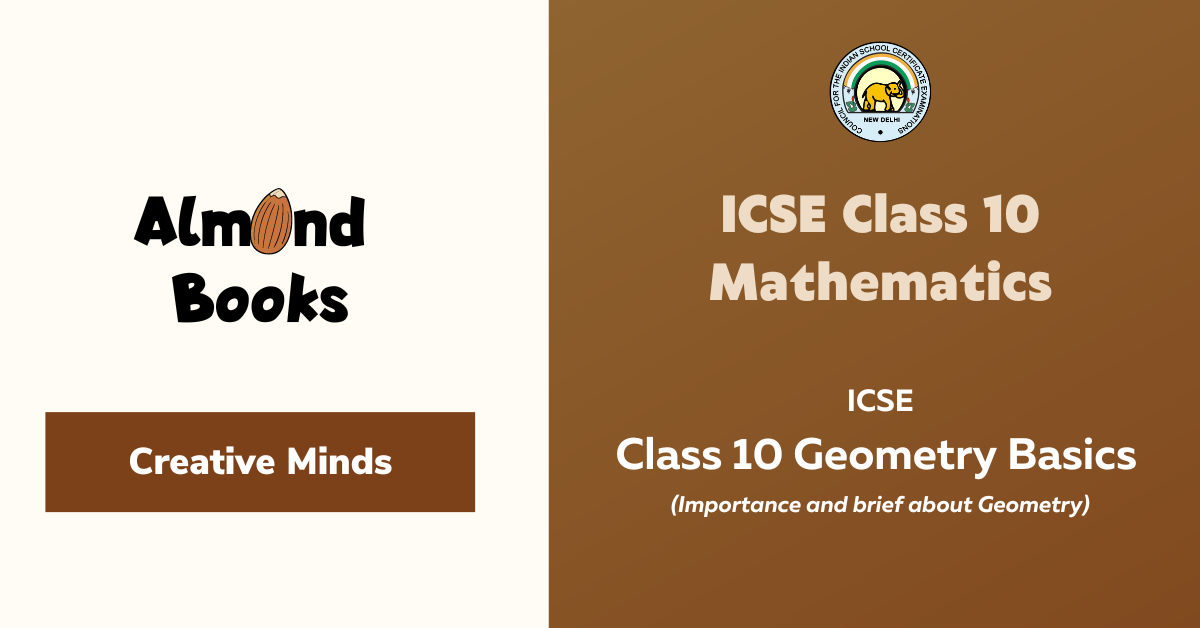Creative Minds

# ICSE Class 10 Geometry Basics

by AlmondBooks Support on Oct 28, 2022

Geometry deals with shapes, angles, dimensions and sizes of a variety of things we see in everyday life.Geometry is derived from Ancient Greek words – ‘Geo’ means ‘Earth’ and ‘metron’ means ‘measurement’.

Basics of Geometry is important for ICSE Class 10. Co-ordinate geometry teaches us the location of a point on a plane.

For Example, the coordinates of a point are (x, y), where x-coordinate (abscissa) denotes the distance of point from the y-axis and y-coordinate (ordinate) denotes the distance of the point from the x-axis.

Basics of Geometry and all the topics for ICSE syllabus for class 10 2023 are included in Almond Books ICSE Class 10 MATHS Study Guide 2022-23 which can be downloaded from the following links:

Almond Books Maths Study Guide for ICSE class 10 (2022-23) Notes + Question Bank + Sample Papers 2023 Exam (Fully Solved) is the best guide students can rely on and to also build up confidence in solving problems. You can buy Now!

It provides students with:

• a strong conceptual knowledge
• Students can explore the key technique in presenting a solution to a problem.
• Detailed explanations and solved papers are provided
• Logically solved step wise answers which help students learn better.

It contains ICSE solved papers for Maths and is based on class 10 syllabus 2023 ICSE which will help the students ace their ICSE exams as it is based on icse 2023 exam pattern.

Tags:
Related Articles